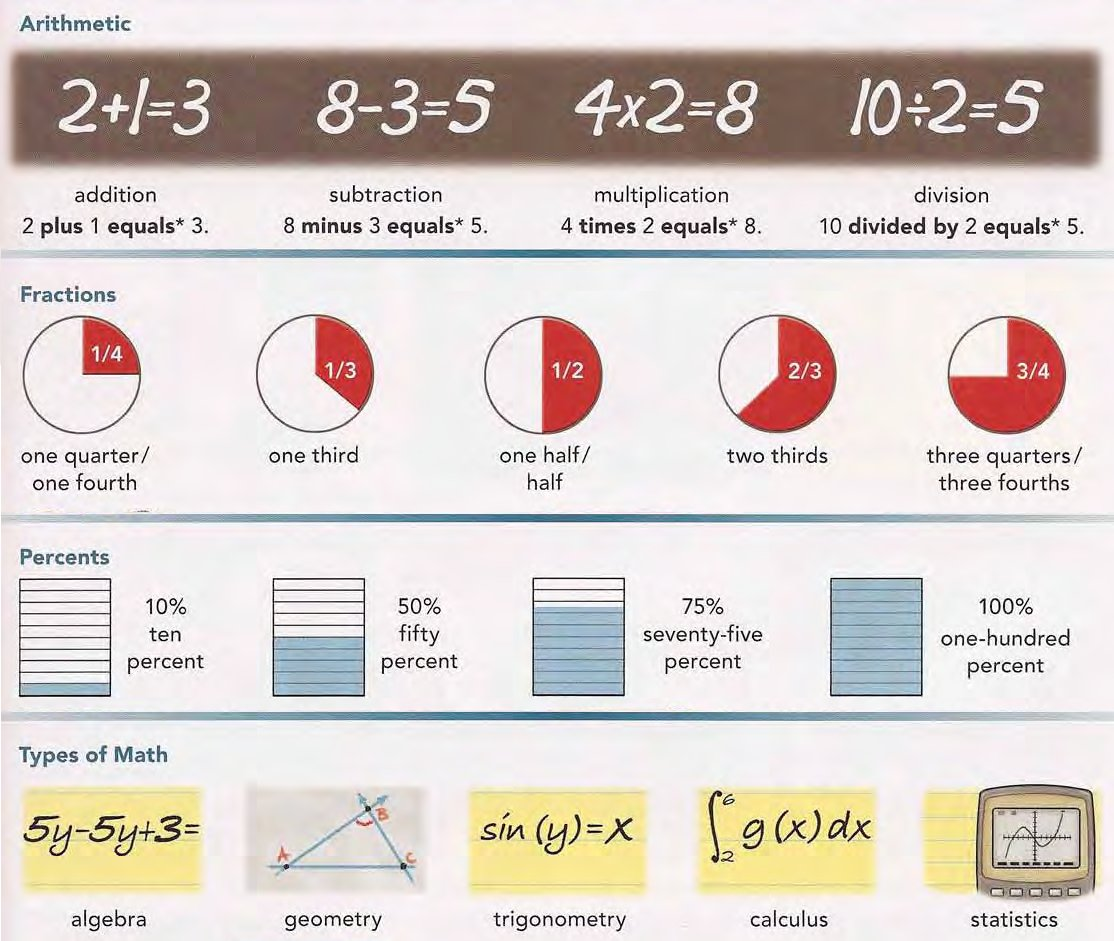Back to index

# MathematicsThere are 26 english words/phrases on this picture:

• 10 divided by 2 equals 5
• ,
• 2 plus 1 equals 3
• ,
• 4 times 2 equals 8
• ,
• 8 minus 3 equals 5
• ,
• ,
• algebra
• ,
• arithmetic
• ,
• calculus
• ,
• division
• ,
• fifty percent
• ,
• fractions
• ,
• geometry
• ,
• multiplication
• ,
• one fourth
• ,
• one half
• ,
• one-hundred percent
• ,
• one third
• ,
• percents
• ,
• seventy-five percent
• ,
• statistics
• ,
• subtraction
• ,
• ten percent
• ,
• three quarters
• ,
• trigonometry
• ,
• two thirds
• ,
• types of math
0 errors